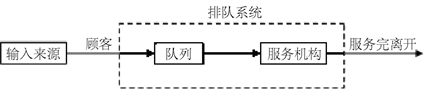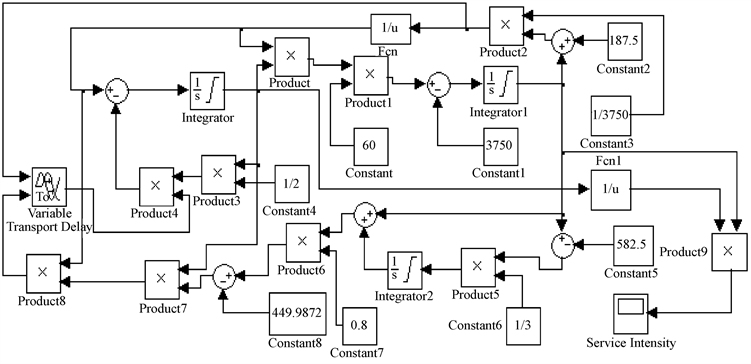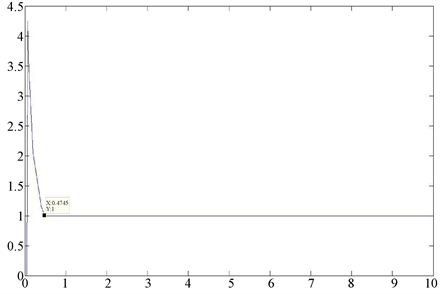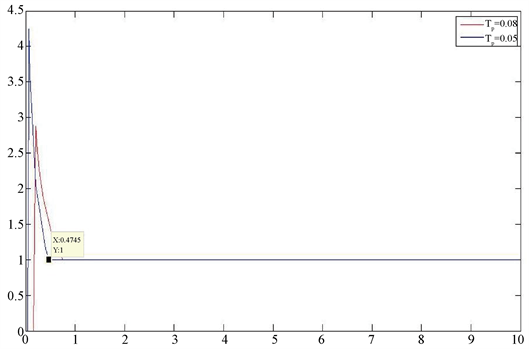﻿ TCP拥塞控制特性在作战指挥网络通信系统中的应用

# TCP拥塞控制特性在作战指挥网络通信系统中的应用Application of TCP Congestion Control Characteristic in Communications System of Operational Command Network

Abstract: Simulink was used to simulate the common non-linear model (AIMD) in TCP congestion control algorithm, and it was applied to the communications system of operational command network. The results showed that the method was feasible, and the performance of the communications system of operational command network could be improved by using TCP congestion control characteristic.

1. 引言

2. 随机服务系统Figure 1. Stochastic service system flow

1) 信息流的依次到达规律，即信息流的抵达过程；

2) 未进入服务系统的信息流的排队规律，即以什么样的排队规律等待随机服务系统的服务；

3) 服务系统(类似于排队系统中处理信息流的节点的数量，以及处理方式等)。

$\lambda$ ——信息流的平均到达率，满足负指数分布，即 $\frac{1}{\lambda }$ 表示信息流的平均到达时间间隔；

$\mu$ ——网络节点的平均处理率，满足负指数分布，即 $\frac{1}{\mu }$ 表示平均处理时间；

$\rho$ ——网络信息流的平均处理强度，即 $\rho =\frac{\lambda }{\mu }$ (对于稳定系统， $\rho \le 1$ )；

$L$ ——信息流的平均长度，即 $L=\frac{\rho }{1-\rho }$ (包括等待处理的信息流数和正在接受处理的信息流数)。

3. TCP拥塞控制模型

$\left\{\begin{array}{l}\left(1\right)\text{\hspace{0.17em}}\text{ }\text{ }\stackrel{˙}{W}\left(t\right)=\frac{1}{R\left(t\right)}-\frac{W\left(t\right)W\left(t-R\left(t\right)\right)}{2R\left(t-R\left(t\right)\right)}p\left(t-R\left(t\right)\right)\\ \left(2\right)\text{\hspace{0.17em}}\text{ }\text{ }\stackrel{˙}{q}\left(t\right)=\frac{W\left(t\right)}{R\left(t\right)}N\left(t\right)-C\\ \left(3\right)\text{\hspace{0.17em}}\text{ }\text{ }R\left(t\right)=\frac{q\left(t\right)}{C}+{T}_{p}\end{array}$

4. TCP拥塞控制特性在随机服务系统中的应用

$\lambda \left(t\right)=\frac{q\left(t\right)}{t}$ ——系统t时刻顾客到达率；

$\mu \left(t\right)=\frac{W\left(t\right)}{t}$ ——系统t时刻服务率；

$\rho \left(t\right)=\frac{\lambda \left(t\right)}{\mu \left(t\right)}$ ——系统t时刻服务强度；

$N\left(t\right)=L\left(t\right)$ ——系统t时刻队长；

(当系统达到稳定时， $N\left(t\right)=L\left(t\right)=N=L=\frac{\stackrel{¯}{\rho \left(t\right)}}{1-\stackrel{¯}{\rho \left(t\right)}}=\frac{\rho }{1-\rho }$ )

${T}_{p}$ ——由于服务窗口拥塞导致的顾客等待时延。

5.1. 仿真环境

Operating System: Microsoft Windows XP Version 5.1 (Build 2600: Service Pack 2)

MATLAB Version: 7.1.0.246 (R14) Service Pack 3Figure 2. Simulink simulates TCP congestion control characteristics

5.2. 仿真结果演示Figure 3. System service intensity

$L\left(t\right)=N\left(t\right)=N=L=\frac{\stackrel{¯}{\rho \left(t\right)}}{1-\stackrel{¯}{\rho \left(t\right)}}=\frac{\rho }{1-\rho }$ (4)Figure 4. System service intensity with different delays

6. 小结

 Tanenbaum, A.S. 计算机网络[M]. 潘爱民, 译. 北京: 清华大学出版社, 2004: 467-472.

 宋振峰, 席志红, 刘飞. 基于Matlab的M/M/m排队模型的仿真[J]. 现代电子技术, 2005, 28(6): 29-30.

 陈鑫林. 现代通信中的排队论[M]. 北京: 电子工业出版社, 1999.

 盛友招. 排队论及其在现代通信中的应用[M]. 北京: 人民邮电出版社, 2007.

 杨丰瑞, 刘辉, 等. 通信网络规划[M]. 北京: 人民邮电出版社, 2005: 77-90.

 叶酉荪, 南庚. 军事通信网分析与系统集成[M]. 北京: 国防工业出版社, 2005: 27-38.

 董希华, 刘峰. TCP-AQM拥塞控制机制的稳定性-时间域方法[J]. 微电子学与计算机, 2003, 20(11): 14-16.

 王德进. 比例-积分TCP/AQM网络稳定性分析的参数空间方法(英文)[J]. 自动化学报, 2007, 33(7): 757-758.

 罗超, 陈宗海, 薛福珍. 基于Simulink的TCP/RED仿真研究[J]. 系统仿真学报, 2004, 16(6): 1163.

 张天魁, 郭彩丽, 曾志民, 冯春燕. 基于Simulink的TCP/PI模型仿真研究[J]. 系统仿真学报, 2006, 18(12): 3554-3555.

 陈相宁, 王京, 程时昕, 等. 多个TCP连接的拥塞丢包模型[J]. 电子学报, 2002, 30(7): 990-994.

Top